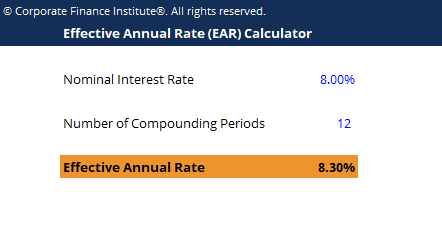# Effective Annual Rate Calculator

## Effective Annual Rate Calculator

This effective annual rate calculator will help you compute the effect annual rate given the nominal interest rate and the number of compounding periods.

This is a screenshot of the effective annual rate calculator:### Download the Free Template

Enter your name and email in the form below and download the free template now!

### Effective Annual Rate Calculator

Download the free Excel template now to advance your finance knowledge!

The Effective Annual Rate (EAR) is the rate of interest actually earned on an investment or paid on a loan as a result of compounding the interest over a given period of time. It is usually higher than the nominal rate and is used to compare different financial products that calculate annual interest with different compounding periods – weekly, monthly, yearly, etc.  Increasing the number of compounding periods makes the effective annual interest rate increase as time goes by.

The effective annual rate is normally higher than the nominal rate because the nominal rate quotes a yearly percentage rate regardless of compounding. Increasing the number of compounding periods increases the effective annual rate as compared to the nominal rate. To spin it in another light, an investment that is compounded annually will have an effective annual rate that is equal to its nominal rate. However, if the same investment was instead compounded quarterly, the effective annual rate would then be higher.

The formula for the EAR is:

Effective Annual Rate = (1 + (nominal interest rate / number of compounding periods)) ^ (number of compounding periods) – 1

### More Free Templates

For more resources, check out our business templates library to download numerous free Excel modeling, PowerPoint presentation and Word document templates.

• Excel Modeling Templates
• PowerPoint Presentation Templates
• Transaction Document Templates

### Financial Analyst Certification

Become a certified Financial Modeling and Valuation Analyst (FMVA)® by completing CFI’s online financial modeling classes and training program!